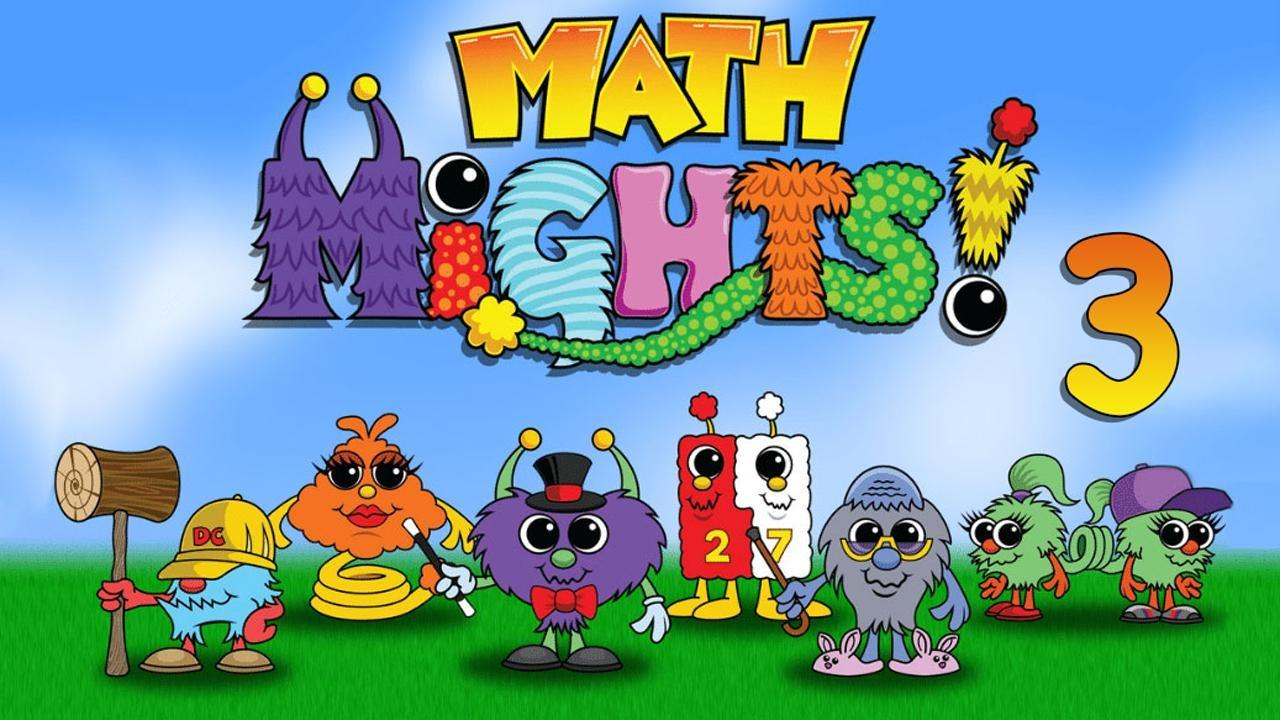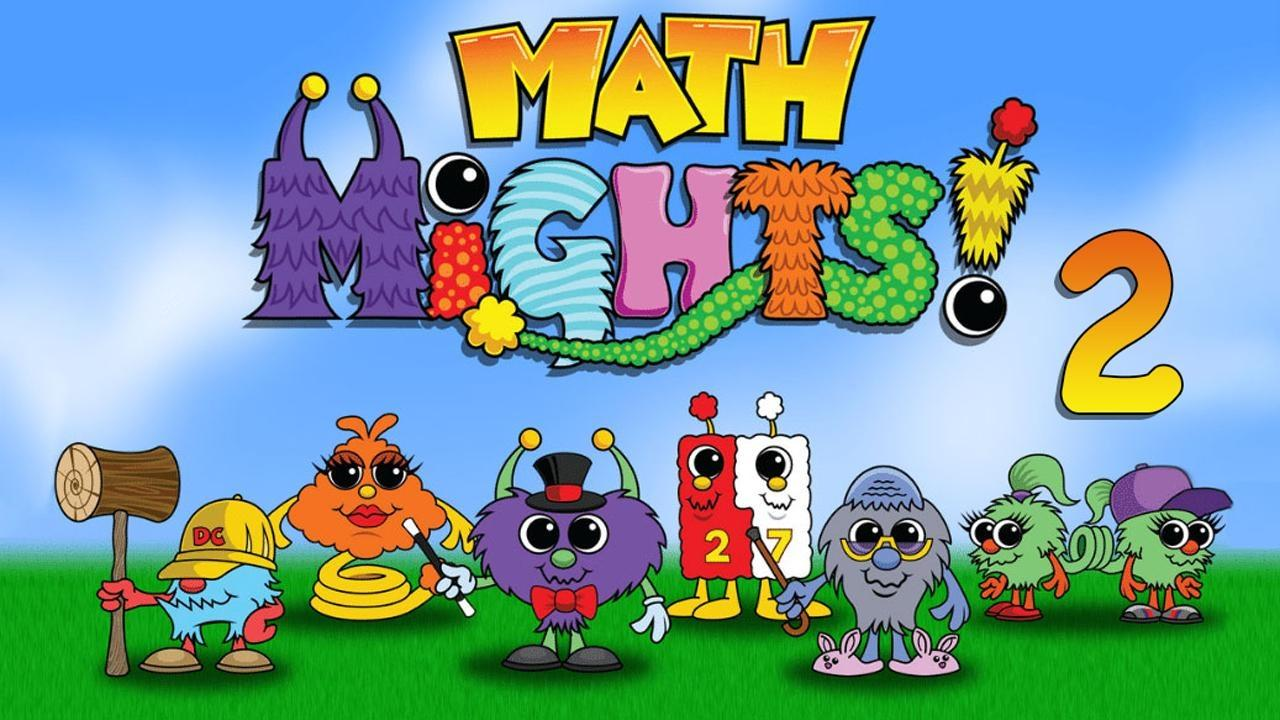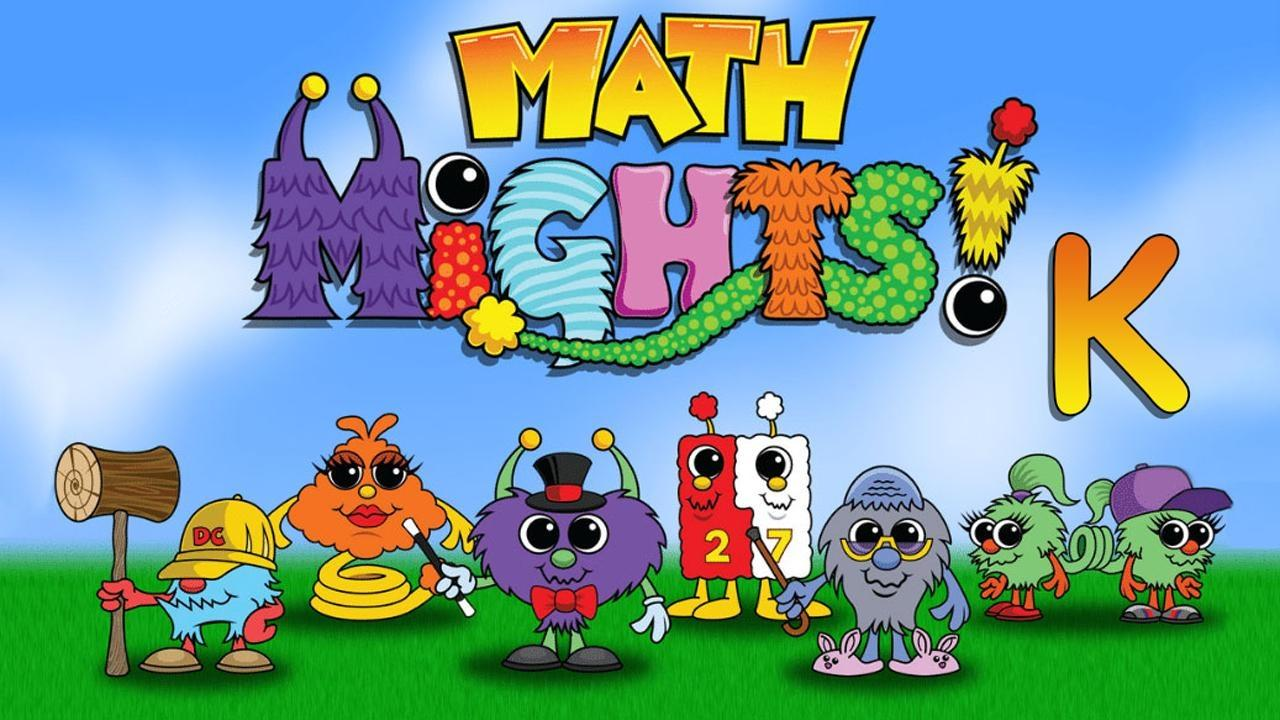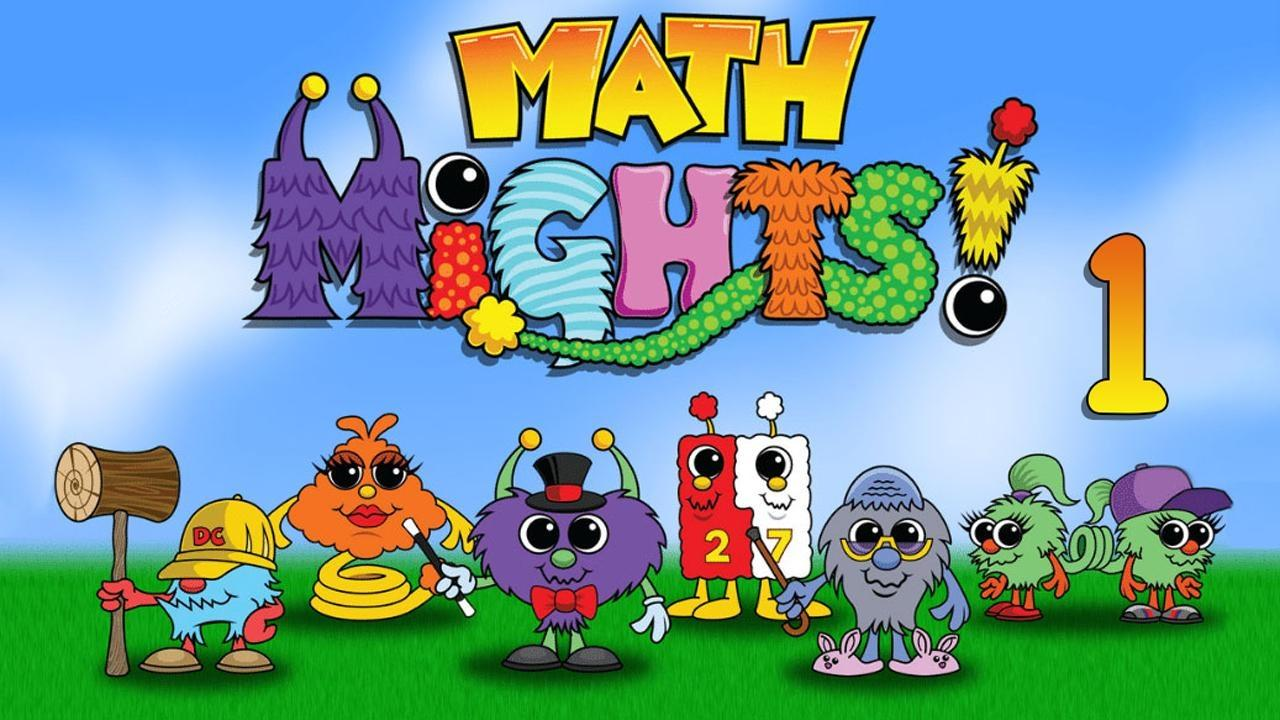# Season 4Math Mights

Collect Data on a Line Plot

Collect data and create our own line plot to represent our data.

### Collect Data on a Line PlotMath Mights

Add and subtract three-digit numbers with Springling and Minni and Subbi.

### Add and Subtract 3-Digit NumbersMath Mights

Write Equations for Numbers 11-19

Using a Rekenrek, work with numbers 11-19, and write equations.

### Write Equations for Numbers 11-19Math Mights

Build New Shapes

Work on making 10 and build new shapes from smaller shapes.

### Build New ShapesMath Mights

Interpret Line Plots

Make sense of line plots with lengths in halves and quarter inches.

### Interpret Line PlotsMath Mights

Find Differences Between Numbers

Work on partial sums and find the difference between numbers with Springling.

### Find Differences Between NumbersMath Mights

Triangles, Rectangles and Squares

Explore what makes a shape a triangle, a rectangle, or a square.

### Triangles, Rectangles and SquaresMath Mights

Numbers 11-19 in different ways

Show numbers 11-19 in different ways using a double ten frame.

### Numbers 11-19 in different waysMath Mights

Measuring with Rulers

Measure length using a ruler marked with halves and quarters of an inch with Ms. Askew.

### Measuring with RulersMath Mights

Sort Flat Shapes

Today, we will sort flat shapes and create a data display to represent our sorts.

### Sort Flat ShapesMath Mights

Add and Subtract 10s or 100s

Join Mrs. McCartney for a Number Talk with Abracus, then add and subtract tens and hundred

### Add and Subtract 10s or 100sMath Mights

Numbers 11-19

Today, we will represent numbers with ten frames and dots or counters.

### Numbers 11-19Math Mights

Solid Shapes

We'll sort, describe and create solid shapes, and talk with Abracus about compensation.

### Solid ShapesMath Mights

10-Frames and More

Work on the double ten frame with Dotson and Mrs. Gray, then compose numbers 11-19.

### 10-Frames and MoreMath Mights

We'll compare numbers and decide to add or subtract with Springling and T-Pops.

### Compare then Add or SubtractMath Mights

Measuring with Halves or Fourths

Join Ms. Askew for a Fraction Number Talk, then measure length in quarters of an inch.